# QPainterPath#

The `QPainterPath` class provides a container for painting operations, enabling graphical shapes to be constructed and reused. More

## Detailed Description#

A painter path is an object composed of a number of graphical building blocks, such as rectangles, ellipses, lines, and curves. Building blocks can be joined in closed subpaths, for example as a rectangle or an ellipse. A closed path has coinciding start and end points. Or they can exist independently as unclosed subpaths, such as lines and curves.

A `QPainterPath` object can be used for filling, outlining, and clipping. To generate fillable outlines for a given painter path, use the `QPainterPathStroker` class. The main advantage of painter paths over normal drawing operations is that complex shapes only need to be created once; then they can be drawn many times using only calls to the `drawPath()` function.

`QPainterPath` provides a collection of functions that can be used to obtain information about the path and its elements. In addition it is possible to reverse the order of the elements using the `toReversed()` function. There are also several functions to convert this painter path object into a polygon representation.

### Composing a QPainterPath#

A `QPainterPath` object can be constructed as an empty path, with a given start point, or as a copy of another `QPainterPath` object. Once created, lines and curves can be added to the path using the `lineTo()` , `arcTo()` , `cubicTo()` and `quadTo()` functions. The lines and curves stretch from the `currentPosition()` to the position passed as argument.

The `currentPosition()` of the `QPainterPath` object is always the end position of the last subpath that was added (or the initial start point). Use the `moveTo()` function to move the `currentPosition()` without adding a component. The `moveTo()` function implicitly starts a new subpath, and closes the previous one. Another way of starting a new subpath is to call the `closeSubpath()` function which closes the current path by adding a line from the `currentPosition()` back to the path’s start position. Note that the new path will have (0, 0) as its initial `currentPosition()` .

`QPainterPath` class also provides several convenience functions to add closed subpaths to a painter path: `addEllipse()` , `addPath()` , `addRect()` , `addRegion()` and `addText()` . The `addPolygon()` function adds an unclosed subpath. In fact, these functions are all collections of `moveTo()` , `lineTo()` and `cubicTo()` operations.

In addition, a path can be added to the current path using the `connectPath()` function. But note that this function will connect the last element of the current path to the first element of given one by adding a line.

Below is a code snippet that shows how a `QPainterPath` object can be used: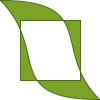```path = QPainterPath() path.addRect(20, 20, 60, 60) path.moveTo(0, 0) path.cubicTo(99, 0, 50, 50, 99, 99) path.cubicTo(0, 99, 50, 50, 0, 0) painter = QPainter(self) painter.fillRect(0, 0, 100, 100, Qt.white) painter.setPen(QPen(QColor(79, 106, 25), 1, Qt.SolidLine, Qt.FlatCap, Qt.MiterJoin)) painter.setBrush(QColor(122, 163, 39)) painter.drawPath(path) ```

The painter path is initially empty when constructed. We first add a rectangle, which is a closed subpath. Then we add two bezier curves which together form a closed subpath even though they are not closed individually. Finally we draw the entire path. The path is filled using the default fill rule, `OddEvenFill` . Qt provides two methods for filling paths:

`OddEvenFill`

`WindingFill`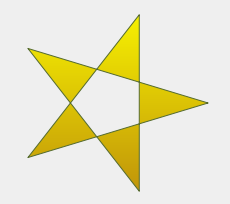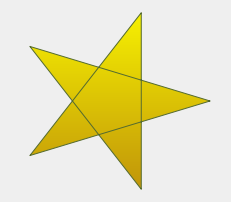See the `FillRule` documentation for the definition of the rules. A painter path’s currently set fill rule can be retrieved using the `fillRule()` function, and altered using the `setFillRule()` function.

### QPainterPath Information#

The `QPainterPath` class provides a collection of functions that returns information about the path and its elements.

The `currentPosition()` function returns the end point of the last subpath that was added (or the initial start point). The `elementAt()` function can be used to retrieve the various subpath elements, the number of elements can be retrieved using the `elementCount()` function, and the `isEmpty()` function tells whether this `QPainterPath` object contains any elements at all.

The `controlPointRect()` function returns the rectangle containing all the points and control points in this path. This function is significantly faster to compute than the exact `boundingRect()` which returns the bounding rectangle of this painter path with floating point precision.

Finally, `QPainterPath` provides the `contains()` function which can be used to determine whether a given point or rectangle is inside the path, and the `intersects()` function which determines if any of the points inside a given rectangle also are inside this path.

### QPainterPath Conversion#

For compatibility reasons, it might be required to simplify the representation of a painter path: `QPainterPath` provides the `toFillPolygon()` , `toFillPolygons()` and `toSubpathPolygons()` functions which convert the painter path into a polygon. The `toFillPolygon()` returns the painter path as one single polygon, while the two latter functions return a list of polygons.

The `toFillPolygons()` and `toSubpathPolygons()` functions are provided because it is usually faster to draw several small polygons than to draw one large polygon, even though the total number of points drawn is the same. The difference between the two is the number of polygons they return: The `toSubpathPolygons()` creates one polygon for each subpath regardless of intersecting subpaths (i.e. overlapping bounding rectangles), while the `toFillPolygons()` functions creates only one polygon for overlapping subpaths.

The `toFillPolygon()` and `toFillPolygons()` functions first convert all the subpaths to polygons, then uses a rewinding technique to make sure that overlapping subpaths can be filled using the correct fill rule. Note that rewinding inserts additional lines in the polygon so the outline of the fill polygon does not match the outline of the path.

### Examples#

Qt provides the Painter Paths Example and the Vector Deformation example which are located in Qt’s example directory.

The Painter Paths Example shows how painter paths can be used to build complex shapes for rendering and lets the user experiment with the filling and stroking. The Vector Deformation Example shows how to use `QPainterPath` to draw text.

Painter Paths Example

Vector Deformation Example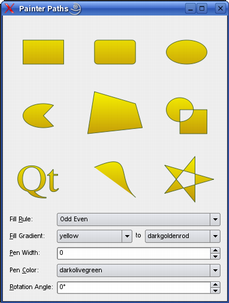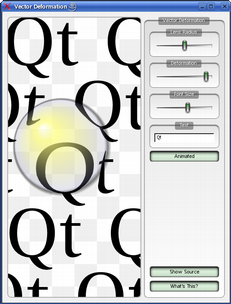class PySide6.QtGui.QPainterPath#

PySide6.QtGui.QPainterPath(other)

PySide6.QtGui.QPainterPath(startPoint)

Parameters

Constructs an empty `QPainterPath` object.

Creates a `QPainterPath` object that is a copy of the given `path`.

`operator=()`

Creates a `QPainterPath` object with the given `startPoint` as its current position.

PySide6.QtGui.QPainterPath.ElementType#

This enum describes the types of elements used to connect vertices in subpaths.

Note that elements added as closed subpaths using the `addEllipse()` , `addPath()` , `addPolygon()` , `addRect()` , `addRegion()` and `addText()` convenience functions, is actually added to the path as a collection of separate elements using the `moveTo()` , `lineTo()` and `cubicTo()` functions.

Constant

Description

QPainterPath.MoveToElement

A new subpath. See also `moveTo()` .

QPainterPath.LineToElement

A line. See also `lineTo()` .

QPainterPath.CurveToElement

A curve. See also `cubicTo()` and `quadTo()` .

QPainterPath.CurveToDataElement

The extra data required to describe a curve in a CurveToElement element.

Parameters
• rx – float

• ry – float

Creates an ellipse positioned at `center` with radii `rx` and `ry`, and adds it to the painter path as a closed subpath.

Parameters
• x – float

• y – float

• w – float

• h – float

Creates an ellipse within the bounding rectangle defined by its top-left corner at (`x`, `y`), `width` and `height`, and adds it to the painter path as a closed subpath.

Parameters

Creates an ellipse within the specified `boundingRectangle` and adds it to the painter path as a closed subpath.

The ellipse is composed of a clockwise curve, starting and finishing at zero degrees (the 3 o’clock position).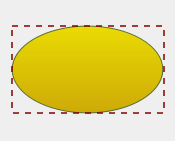```myGradient = QLinearGradient() myPen = QPen() boundingRectangle = QRectF() myPath = QPainterPath() myPath.addEllipse(boundingRectangle) painter = QPainter(self) painter.setBrush(myGradient) painter.setPen(myPen) painter.drawPath(myPath) ```

`arcTo()` `drawEllipse()` `Composing a QPainterPath`

Parameters

Adds the given `path` to this path as a closed subpath.

`connectPath()` `Composing a QPainterPath`

Parameters

Adds the given `polygon` to the path as an (unclosed) subpath.

Note that the current position after the polygon has been added, is the last point in `polygon`. To draw a line back to the first point, use the `closeSubpath()` function.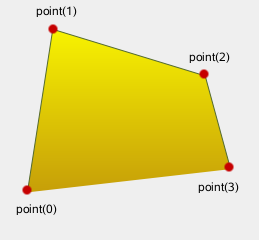```myGradient = QLinearGradient() myPen = QPen() myPolygon = QPolygonF() myPath = QPainterPath() myPath.addPolygon(myPolygon) painter = QPainter(self) painter.setBrush(myGradient) painter.setPen(myPen) painter.drawPath(myPath) ```

`lineTo()` `Composing a QPainterPath`

Parameters

Adds the given `rectangle` to this path as a closed subpath.

The `rectangle` is added as a clockwise set of lines. The painter path’s current position after the `rectangle` has been added is at the top-left corner of the rectangle.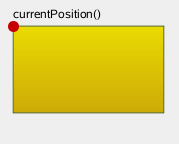```myGradient = QLinearGradient() myPen = QPen() myRectangle = QRectF() myPath = QPainterPath() myPath.addRect(myRectangle) painter = QPainter(self) painter.setBrush(myGradient) painter.setPen(myPen) painter.drawPath(myPath) ```

`addRegion()` `lineTo()` `Composing a QPainterPath`

Parameters
• x – float

• y – float

• w – float

• h – float

Adds a rectangle at position (`x`, `y`), with the given `width` and `height`, as a closed subpath.

Parameters

Adds the given `region` to the path by adding each rectangle in the region as a separate closed subpath.

`addRect()` `Composing a QPainterPath`

Parameters

Adds the given rectangle `rect` with rounded corners to the path.

The `xRadius` and `yRadius` arguments specify the radii of the ellipses defining the corners of the rounded rectangle. When `mode` is `RelativeSize` , `xRadius` and `yRadius` are specified in percentage of half the rectangle’s width and height respectively, and should be in the range 0.0 to 100.0.

Parameters
• x – float

• y – float

• w – float

• h – float

• mode`SizeMode`

Adds the given rectangle `x`, `y`, `w`, `h` with rounded corners to the path.

Parameters
• text – str

Adds the given `text` to this path as a set of closed subpaths created from the `font` supplied. The subpaths are positioned so that the left end of the text’s baseline lies at the specified `point`.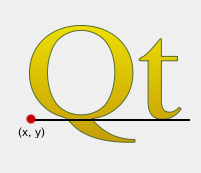```myGradient = QLinearGradient() myPen = QPen() myFont = QFont() baseline = QPointF(x, y) myPath = QPainterPath() myPath.addText(baseline, myFont, tr("Qt")) painter = QPainter(self) painter.setBrush(myGradient) painter.setPen(myPen) painter.drawPath(myPath) ```

`drawText()` `Composing a QPainterPath`

Parameters
• x – float

• y – float

• text – str

Adds the given `text` to this path as a set of closed subpaths created from the `font` supplied. The subpaths are positioned so that the left end of the text’s baseline lies at the point specified by (`x`, `y`).

PySide6.QtGui.QPainterPath.angleAtPercent(t)#
Parameters

t – float

Return type

float

Returns the angle of the path tangent at the percentage `t`. The argument `t` has to be between 0 and 1.

Positive values for the angles mean counter-clockwise while negative values mean the clockwise direction. Zero degrees is at the 3 o’clock position.

Note that similarly to the other percent methods, the percentage measurement is not linear with regards to the length if curves are present in the path. When curves are present the percentage argument is mapped to the t parameter of the Bezier equations.

PySide6.QtGui.QPainterPath.arcMoveTo(rect, angle)#
Parameters
• angle – float

Creates a move to that lies on the arc that occupies the given `rectangle` at `angle`.

Angles are specified in degrees. Clockwise arcs can be specified using negative angles.

PySide6.QtGui.QPainterPath.arcMoveTo(x, y, w, h, angle)
Parameters
• x – float

• y – float

• w – float

• h – float

• angle – float

Creates a move to that lies on the arc that occupies the `QRectF` (`x`, `y`, `width`, `height`) at `angle`.

PySide6.QtGui.QPainterPath.arcTo(rect, startAngle, arcLength)#
Parameters
• startAngle – float

• arcLength – float

Creates an arc that occupies the given `rectangle`, beginning at the specified `startAngle` and extending `sweepLength` degrees counter-clockwise.

Angles are specified in degrees. Clockwise arcs can be specified using negative angles.

Note that this function connects the starting point of the arc to the current position if they are not already connected. After the arc has been added, the current position is the last point in arc. To draw a line back to the first point, use the `closeSubpath()` function.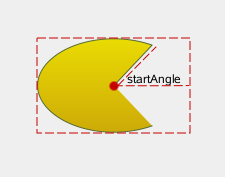```myPath = QPainterPath() myPath.moveTo(center) myPath.arcTo(boundingRect, startAngle, sweepLength) painter = QPainter(self) painter.setBrush(myGradient) painter.setPen(myPen) painter.drawPath(myPath) ```
PySide6.QtGui.QPainterPath.arcTo(x, y, w, h, startAngle, arcLength)
Parameters
• x – float

• y – float

• w – float

• h – float

• startAngle – float

• arcLength – float

Creates an arc that occupies the rectangle `QRectF` (`x`, `y`, `width`, `height`), beginning at the specified `startAngle` and extending `sweepLength` degrees counter-clockwise.

PySide6.QtGui.QPainterPath.boundingRect()#
Return type

`PySide6.QtCore.QRectF`

Returns the bounding rectangle of this painter path as a rectangle with floating point precision.

PySide6.QtGui.QPainterPath.capacity()#
Return type

int

Returns the number of elements allocated by the `QPainterPath` .

PySide6.QtGui.QPainterPath.clear()#

Clears the path elements stored.

This allows the path to reuse previous memory allocations.

PySide6.QtGui.QPainterPath.closeSubpath()#

Closes the current subpath by drawing a line to the beginning of the subpath, automatically starting a new path. The current point of the new path is (0, 0).

If the subpath does not contain any elements, this function does nothing.

`moveTo()` `Composing a QPainterPath`

PySide6.QtGui.QPainterPath.connectPath(path)#
Parameters

Connects the given `path` to this path by adding a line from the last element of this path to the first element of the given path.

`addPath()` `Composing a QPainterPath`

PySide6.QtGui.QPainterPath.contains(p)#
Parameters
Return type

bool

Returns `true` if the given path `p` is contained within the current path. Returns `false` if any edges of the current path and `p` intersect.

Set operations on paths will treat the paths as areas. Non-closed paths will be treated as implicitly closed.

PySide6.QtGui.QPainterPath.contains(pt)
Parameters
Return type

bool

Returns `true` if the given `point` is inside the path, otherwise returns `false`.

PySide6.QtGui.QPainterPath.contains(rect)
Parameters
Return type

bool

Returns `true` if the given `rectangle` is inside the path, otherwise returns `false`.

PySide6.QtGui.QPainterPath.controlPointRect()#
Return type

`PySide6.QtCore.QRectF`

Returns the rectangle containing all the points and control points in this path.

This function is significantly faster to compute than the exact `boundingRect()` , and the returned rectangle is always a superset of the rectangle returned by `boundingRect()` .

PySide6.QtGui.QPainterPath.cubicTo(ctrlPt1, ctrlPt2, endPt)#
Parameters

Adds a cubic Bezier curve between the current position and the given `endPoint` using the control points specified by `c1`, and `c2`.

After the curve is added, the current position is updated to be at the end point of the curve.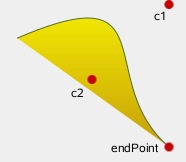```myGradient = QLinearGradient() myPen = QPen() myPath = QPainterPath() myPath.cubicTo(c1, c2, endPoint) painter = QPainter(self) painter.setBrush(myGradient) painter.setPen(myPen) painter.drawPath(myPath) ```

`quadTo()` `Composing a QPainterPath`

PySide6.QtGui.QPainterPath.cubicTo(ctrlPt1x, ctrlPt1y, ctrlPt2x, ctrlPt2y, endPtx, endPty)
Parameters
• ctrlPt1x – float

• ctrlPt1y – float

• ctrlPt2x – float

• ctrlPt2y – float

• endPtx – float

• endPty – float

Adds a cubic Bezier curve between the current position and the end point (`endPointX`, `endPointY`) with control points specified by (`c1X`, `c1Y`) and (`c2X`, `c2Y`).

PySide6.QtGui.QPainterPath.currentPosition()#
Return type

`PySide6.QtCore.QPointF`

Returns the current position of the path.

PySide6.QtGui.QPainterPath.elementAt(i)#
Parameters

i – int

Return type

`PySide6.QtGui.QPainterPath.Element`

Returns the element at the given `index` in the painter path.

`ElementType` `elementCount()` `isEmpty()`

PySide6.QtGui.QPainterPath.elementCount()#
Return type

int

Returns the number of path elements in the painter path.

`ElementType` `elementAt()` `isEmpty()`

PySide6.QtGui.QPainterPath.fillRule()#
Return type

`FillRule`

Returns the painter path’s currently set fill rule.

PySide6.QtGui.QPainterPath.intersected(r)#
Parameters
Return type

`PySide6.QtGui.QPainterPath`

Returns a path which is the intersection of this path’s fill area and `p`'s fill area. Bezier curves may be flattened to line segments due to numerical instability of doing bezier curve intersections.

PySide6.QtGui.QPainterPath.intersects(rect)#
Parameters
Return type

bool

Returns `true` if any point in the given `rectangle` intersects the path; otherwise returns `false`.

There is an intersection if any of the lines making up the rectangle crosses a part of the path or if any part of the rectangle overlaps with any area enclosed by the path. This function respects the current `fillRule` to determine what is considered inside the path.

PySide6.QtGui.QPainterPath.intersects(p)
Parameters
Return type

bool

Returns `true` if the current path intersects at any point the given path `p`. Also returns `true` if the current path contains or is contained by any part of `p`.

Set operations on paths will treat the paths as areas. Non-closed paths will be treated as implicitly closed.

PySide6.QtGui.QPainterPath.isEmpty()#
Return type

bool

Returns `true` if either there are no elements in this path, or if the only element is a `MoveToElement` ; otherwise returns `false`.

PySide6.QtGui.QPainterPath.length()#
Return type

float

Returns the length of the current path.

PySide6.QtGui.QPainterPath.lineTo(p)#
Parameters

Adds a straight line from the current position to the given `endPoint`. After the line is drawn, the current position is updated to be at the end point of the line.

`addPolygon()` `addRect()` `Composing a QPainterPath`

PySide6.QtGui.QPainterPath.lineTo(x, y)
Parameters
• x – float

• y – float

Draws a line from the current position to the point (`x`, `y`).

PySide6.QtGui.QPainterPath.moveTo(p)#
Parameters

Moves the current point to the given `point`, implicitly starting a new subpath and closing the previous one.

`closeSubpath()` `Composing a QPainterPath`

PySide6.QtGui.QPainterPath.moveTo(x, y)
Parameters
• x – float

• y – float

Moves the current position to (`x`, `y`) and starts a new subpath, implicitly closing the previous path.

PySide6.QtGui.QPainterPath.__ne__(other)#
Parameters
Return type

bool

Returns `true` if this painter path differs from the given `path`.

Note that comparing paths may involve a per element comparison which can be slow for complex paths.

`operator==()`

PySide6.QtGui.QPainterPath.__and__(other)#
Parameters
Return type

`PySide6.QtGui.QPainterPath`

Returns the intersection of this path and the `other` path.

`intersected()` `operator&=()` `united()` `operator|()`

PySide6.QtGui.QPainterPath.__iand__(other)#
Parameters
Return type

`PySide6.QtGui.QPainterPath`

Intersects this path with `other` and returns a reference to this path.

`intersected()` `operator&()` `operator|=()`

PySide6.QtGui.QPainterPath.__mul__(m)#
Parameters
Return type

`PySide6.QtGui.QPainterPath`

Parameters
Return type

`PySide6.QtGui.QPainterPath`

Returns the union of this path and the `other` path. This function is equivalent to operator|().

`united()` `operator+=()` `operator-()`

Parameters
Return type

`PySide6.QtGui.QPainterPath`

Unites this path with `other`, and returns a reference to this path. This is equivalent to operator|=().

`united()` `operator+()` `operator-=()`

PySide6.QtGui.QPainterPath.__sub__(other)#
Parameters
Return type

`PySide6.QtGui.QPainterPath`

Subtracts the `other` path from a copy of this path, and returns the copy.

`subtracted()` `operator-=()` `operator+()`

PySide6.QtGui.QPainterPath.__isub__(other)#
Parameters
Return type

`PySide6.QtGui.QPainterPath`

Subtracts `other` from this path, and returns a reference to this path.

`subtracted()` `operator-()` `operator+=()`

PySide6.QtGui.QPainterPath.__eq__(other)#
Parameters
Return type

bool

Returns `true` if this painterpath is equal to the given `path`.

Note that comparing paths may involve a per element comparison which can be slow for complex paths.

`operator!=()`

PySide6.QtGui.QPainterPath.__or__(other)#
Parameters
Return type

`PySide6.QtGui.QPainterPath`

Returns the union of this path and the `other` path.

`united()` `operator|=()` `intersected()` `operator&()`

PySide6.QtGui.QPainterPath.__ior__(other)#
Parameters
Return type

`PySide6.QtGui.QPainterPath`

Unites this path with `other` and returns a reference to this path.

`united()` `operator|()` `operator&=()`

PySide6.QtGui.QPainterPath.percentAtLength(t)#
Parameters

t – float

Return type

float

Returns percentage of the whole path at the specified length `len`.

Note that similarly to other percent methods, the percentage measurement is not linear with regards to the length, if curves are present in the path. When curves are present the percentage argument is mapped to the t parameter of the Bezier equations.

PySide6.QtGui.QPainterPath.pointAtPercent(t)#
Parameters

t – float

Return type

`PySide6.QtCore.QPointF`

Returns the point at at the percentage `t` of the current path. The argument `t` has to be between 0 and 1.

Note that similarly to other percent methods, the percentage measurement is not linear with regards to the length, if curves are present in the path. When curves are present the percentage argument is mapped to the t parameter of the Bezier equations.

Parameters

Adds a quadratic Bezier curve between the current position and the given `endPoint` with the control point specified by `c`.

After the curve is added, the current point is updated to be at the end point of the curve.

`cubicTo()` `Composing a QPainterPath`

Parameters
• ctrlPtx – float

• ctrlPty – float

• endPtx – float

• endPty – float

Adds a quadratic Bezier curve between the current point and the endpoint (`endPointX`, `endPointY`) with the control point specified by (`cx`, `cy`).

PySide6.QtGui.QPainterPath.reserve(size)#
Parameters

size – int

Reserves a given amount of elements in `QPainterPath` ‘s internal memory.

Attempts to allocate memory for at least `size` elements.

`clear()` `capacity()` `reserve()`

PySide6.QtGui.QPainterPath.setElementPositionAt(i, x, y)#
Parameters
• i – int

• x – float

• y – float

Sets the x and y coordinate of the element at index `index` to `x` and `y`.

PySide6.QtGui.QPainterPath.setFillRule(fillRule)#
Parameters

fillRule`FillRule`

Sets the fill rule of the painter path to the given `fillRule`. Qt provides two methods for filling paths:

`OddEvenFill` (default)

`WindingFill`PySide6.QtGui.QPainterPath.simplified()#
Return type

`PySide6.QtGui.QPainterPath`

Returns a simplified version of this path. This implies merging all subpaths that intersect, and returning a path containing no intersecting edges. Consecutive parallel lines will also be merged. The simplified path will always use the default fill rule, `OddEvenFill` . Bezier curves may be flattened to line segments due to numerical instability of doing bezier curve intersections.

PySide6.QtGui.QPainterPath.slopeAtPercent(t)#
Parameters

t – float

Return type

float

Returns the slope of the path at the percentage `t`. The argument `t` has to be between 0 and 1.

Note that similarly to other percent methods, the percentage measurement is not linear with regards to the length, if curves are present in the path. When curves are present the percentage argument is mapped to the t parameter of the Bezier equations.

PySide6.QtGui.QPainterPath.subtracted(r)#
Parameters
Return type

`PySide6.QtGui.QPainterPath`

Returns a path which is `p`'s fill area subtracted from this path’s fill area.

Set operations on paths will treat the paths as areas. Non-closed paths will be treated as implicitly closed. Bezier curves may be flattened to line segments due to numerical instability of doing bezier curve intersections.

PySide6.QtGui.QPainterPath.swap(other)#
Parameters

Swaps painter path `other` with this painter path. This operation is very fast and never fails.

PySide6.QtGui.QPainterPath.toFillPolygon([matrix=QTransform()])#
Parameters
Return type

`PySide6.QtGui.QPolygonF`

Converts the path into a polygon using the `QTransform` `matrix`, and returns the polygon.

The polygon is created by first converting all subpaths to polygons, then using a rewinding technique to make sure that overlapping subpaths can be filled using the correct fill rule.

Note that rewinding inserts addition lines in the polygon so the outline of the fill polygon does not match the outline of the path.

`toSubpathPolygons()` `toFillPolygons()` `QPainterPath Conversion`

PySide6.QtGui.QPainterPath.toFillPolygons([matrix=QTransform()])#
Parameters
Return type

Converts the path into a list of polygons using the `QTransform` `matrix`, and returns the list.

The function differs from the `toFillPolygon()` function in that it creates several polygons. It is provided because it is usually faster to draw several small polygons than to draw one large polygon, even though the total number of points drawn is the same.

The toFillPolygons() function differs from the `toSubpathPolygons()` function in that it create only polygon for subpaths that have overlapping bounding rectangles.

Like the `toFillPolygon()` function, this function uses a rewinding technique to make sure that overlapping subpaths can be filled using the correct fill rule. Note that rewinding inserts addition lines in the polygons so the outline of the fill polygon does not match the outline of the path.

`toSubpathPolygons()` `toFillPolygon()` `QPainterPath Conversion`

PySide6.QtGui.QPainterPath.toReversed()#
Return type

`PySide6.QtGui.QPainterPath`

Creates and returns a reversed copy of the path.

It is the order of the elements that is reversed: If a `QPainterPath` is composed by calling the `moveTo()` , `lineTo()` and `cubicTo()` functions in the specified order, the reversed copy is composed by calling `cubicTo()` , `lineTo()` and `moveTo()` .

PySide6.QtGui.QPainterPath.toSubpathPolygons([matrix=QTransform()])#
Parameters
Return type

Converts the path into a list of polygons using the `QTransform` `matrix`, and returns the list.

This function creates one polygon for each subpath regardless of intersecting subpaths (i.e. overlapping bounding rectangles). To make sure that such overlapping subpaths are filled correctly, use the `toFillPolygons()` function instead.

`toFillPolygons()` `toFillPolygon()` `QPainterPath Conversion`

PySide6.QtGui.QPainterPath.translate(offset)#
Parameters

Translates all elements in the path by the given `offset`.

PySide6.QtGui.QPainterPath.translate(dx, dy)
Parameters
• dx – float

• dy – float

Translates all elements in the path by (`dx`, `dy`).

PySide6.QtGui.QPainterPath.translated(offset)#
Parameters
Return type

`PySide6.QtGui.QPainterPath`

Returns a copy of the path that is translated by the given `offset`.

PySide6.QtGui.QPainterPath.translated(dx, dy)
Parameters
• dx – float

• dy – float

Return type

`PySide6.QtGui.QPainterPath`

Returns a copy of the path that is translated by (`dx`, `dy`).

PySide6.QtGui.QPainterPath.united(r)#
Parameters
Return type

`PySide6.QtGui.QPainterPath`

Returns a path which is the union of this path’s fill area and `p`'s fill area.

Set operations on paths will treat the paths as areas. Non-closed paths will be treated as implicitly closed. Bezier curves may be flattened to line segments due to numerical instability of doing bezier curve intersections.